### Introduction

This CFD simulation is inspired by the experimental work of J.S. Jayakumar et al. . The physical apparatus tested was a shell and tube heat exchanger used frequently by industry to transfer energy from one fluid to another. The geometry of this experimental apparatus is shown in Figures 1-2. Figure 1 details the geometry of a small tube that is coiled in a circular arrangement inside a shell, Figure 2. The inside diameter of the stainless steel tube is 10 mm with a coil diameter of 300 mm, pitch of 30 mm and a tube wall thickness of 2.7 mm. The shell is made of stainless steel and insulated. Two separate fluid flow paths are present: one inside the tube, the second outside the tube (inside the shell). Water was used as the working fluid in the experiment, where hot water was provided to the tube at a constant temperature of 360 K and cool water to the shell at 300 K. The outlet water temperatures of both the shell and tube were measured, allowing the overall heat transfer coefficient to be calculated.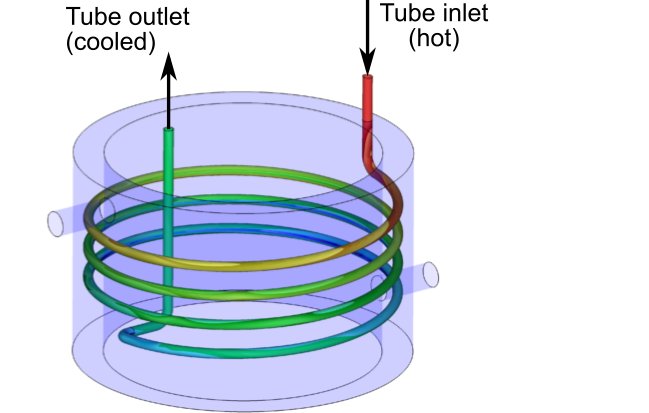Figure 1 (surface temperature)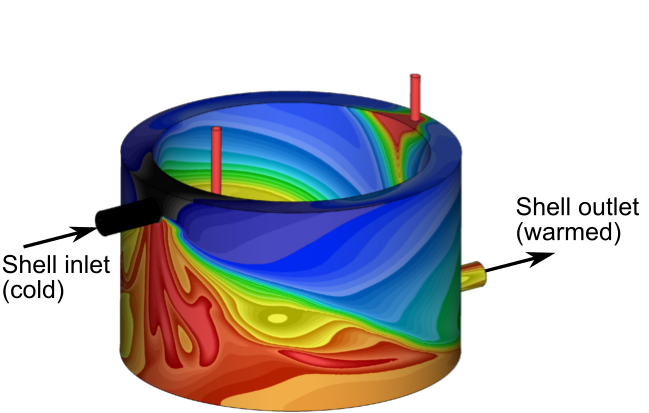Figure 2 (surface temperature)

Simulation of this experimental setup highlights the following Azore® capabilities:

Multiple Fluid Paths – Azore can accommodate many different fluid types, each in separate flow paths. For this problem, the fluids are the same (water), but the fluid paths are only connected to one another through heat transfer.

Temperature Dependent Properties – Fluid properties can be easily defined as constant or dependent on temperature, where temperature dependence can be either piecewise linear or polynomial.

Conjugate heat transfer – The heat path for this problem involves moving energy from the fluid inside the tube through the material of the tube wall and then into the fluid inside the shell. This process is handled automatically by Azore where the tube thickness and material properties are all included in the heat transfer process.

Turbulence – The Reynolds numbers present in this experiment are large enough that the standard K-epsilon turbulence model is utilized.

Mesh Topology – To properly resolve the physics of this problem, it is best to use a hybrid mesh topology composed of a swept hexahedral mesh on the interior of the tube and an unstructured mesh in the shell. Azore can handle control volume mesh topology composed of both traditional cells such as hexahedral and tetrahedral as well as fully polyhedral topologies.

### Model Setup

The geometry of the model domain is shown in Figure 1. This geometry is based on the experimental test section schematic and includes a short inlet and exit tube section before and after the coiled tube region, which is used to create the desired Dean flow (a flow pattern that helps promote heat transfer). The thickness of the tube was not included in the simulation (treated as a thin wall), but the effective thermal thickness and conductivity was included in the simulation. Fluid properties for viscosity, density, thermal conductivity, and specific heat were assumed to vary with water temperature. The standard polynomial form for these properties was used:

fluid property = a + bT +cT2 + dT3+eT4+fT5

The standard K-epsilon model was used along with the wall functions for boundary layer closure. A uniform constant velocity was assumed at the inlet of the tube and the shell flow paths. The inlet turbulent kinetic energy was taken as 10% of the mean velocity and the turbulent dissipation value was determined based on the inlet hydraulic diameter. A constant pressure on the exit of the tube and shell flow paths was assumed.

The model domain was meshed with a combination of hexahedral and polyhedral cells with a total count of 25.6 million. Figure 3 details the internal topology for the mesh. The left most image shows the overall mesh topology and density through half of the model domain. The middle image details the mesh in a cross section through the coiled tube and the right image shows the swept hexahedral mesh inside the tube. Note that the hexahedral mesh and boundary layer cell topologies are used to improve the predictions of the secondary flow structures (Dean flow) inside the tube.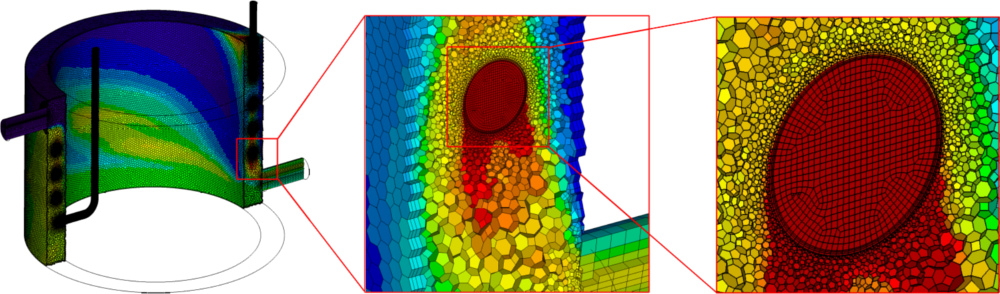Figure 3

### Model Results

The temperature differential between the two flow paths is the main driving force that transfers energy from the warm water inside the tube to the cooler water inside the shell. Heat exchanger designers are well aware of this and typically incorporate features in their heat exchanger designs that maximize the temperature differentials between the two working fluids. For this experimental setup, the temperature differential is improved by the coiled flow path of the tube. The fluid is constantly turning, creating a flow structure that forces the fluid at the center of the tube toward the outside tube surface. This fluid motion maximizes the temperature differential and hence the overall heat transfer efficiency.

Figure 1 shows the tube surface temperature predictions and Figure 2 shows the shell surface temperatures. Figure 4 details a cutting plane through the center of the heat exchanger, characterizing the fluid temperatures inside the experimental fixture. On the right hand side of Figure 4, a zoomed-in view of the flow structure inside the coiled tube is shown. The colors represent the water temperature and the arrows represent the secondary flow motion. Note that the secondary flow is moving the warmer fluid toward the outside surface of the tube.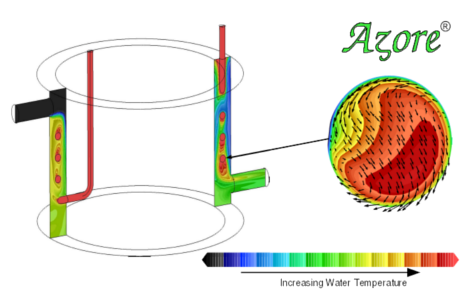Figure 4

This case has been taken from the Verification and Validation test suite of Azore. Figure 5 details the simulation predictions compared to the experimental data collected.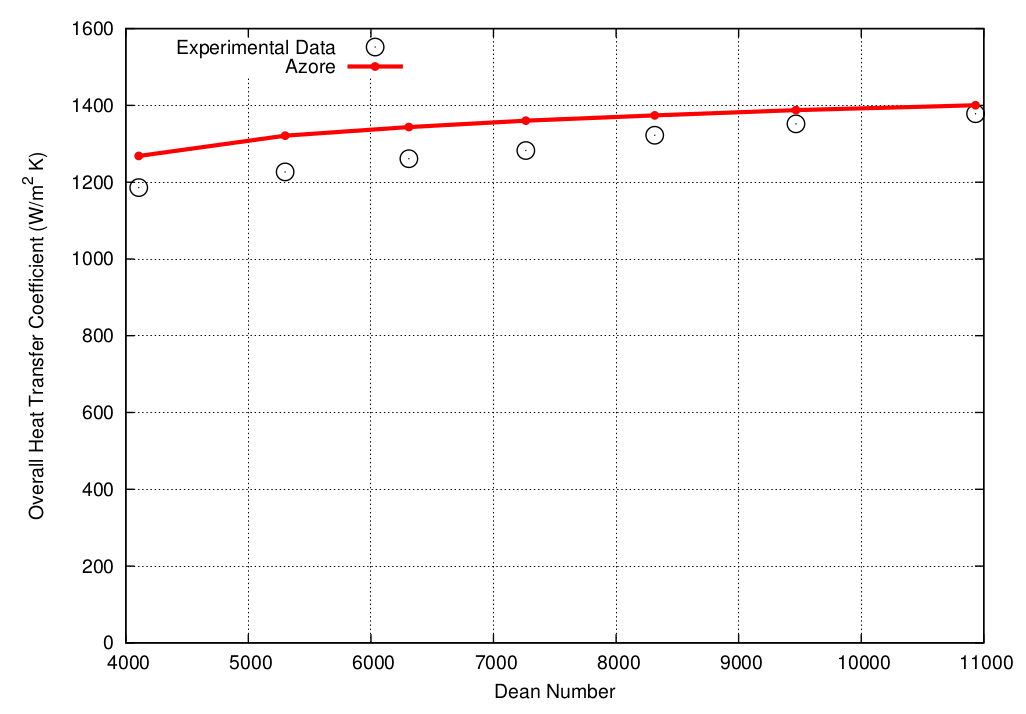Figure 5

 J.S. Jayakumar, S.M. Mahajani, J.C. Mandal, P.K. Vijayan, and Rohidas Bhoi, Experimental and CFD estimation of heat transfer in a helically coiled heat exchangers, Chemical Engineering Research and Design, Volume 86, 2008, pages 221-232.